Error: division by zero

In mathematics, division by zero is not allowed. In Maple, an attempt to divide by zero produces this error.

> sin(0)/0;

Error, division by zero

In some cases when a division by zero results from evaluating some expression at a certain value of a variable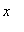, what is really wanted instead is the limit of the expression asapproaches this value. This may be obtained by using limit .

> limit(sin(x)/x, x=0);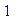Sometimes a division by zero can result when Maple fails to recognize early enough that some quantity is 0.

> series(p/((1-sin(x-p)^2 - cos(x+p)^2)), p = 0,3);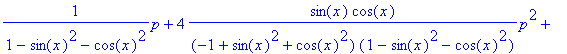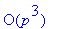> simplify(");

Error, (in simplify/trig) division by zero

When this occurs in series and related commands, you may be able to fix the problem by changing the environment variable Testzero, whose value is the procedure used in series to determine when the leading coefficient is 0. By default this just uses normal .

> eval(Testzero);You can replace normal by simplify to get a stronger test.

> Testzero:= proc(O) evalb(simplify(O)=0) end:

Since series has the remember option, if you try the same series command again it will just remember the value it computed before. To get it to try again, you can make it forget the past values.

> series(p/((1-sin(x-p)^2 - cos(x+p)^2)), p = 0,3);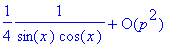There is also a bug in Release 4 that causes this error to occur in some integrals involving products of half-integer powers of linear terms.

> int((2*x+1)^(-3/2)*x^(1/2),x=0..1);

Error, (in int) division by zero

A work-around is to express the integrand as a single square root.

> F:= int(((2*x+1)^(-3)*x)^(1/2),x=0..1);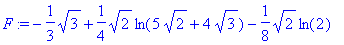See also: forget , int , Integrals involving fractional powers , limit , normal , series , simplify , Testzero

Maple Advisor Database R. Israel, 1998